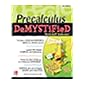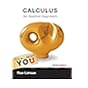Normal view MARC view ISBD view

# Precalculus demystified / Rhonda Huettenmueller

Material type:BookSeries: Publisher: New York : McGraw-Hill, Copyright date: ©2012Edition: Second edition.Description: ix, 534 pages : illustrations ; 24cm. (Demystified).Content type: text Media type: unmediated Carrier type: volumeISBN: 9780071778497 (paperback).
Contents:
Machine generated contents note: Ch 1 Lines -- The slope of a line; Equations of lines; Applications of lines -- Ch 2 Introduction to Functions -- Function Evaluation; More function evaluation; The domain and range of a function -- Ch 3 Functions and Their Graphs -- Reading a graph; Average rate of change; Even/odd functions; Graphing functions with a calculator -- Ch 4 Combinations of Functions -- Arithmetic combinations; Composition of functions; 1-1 functions and their inverses -- Ch 5 Transformations of a Function -- Families of functions; Shifting graphs; Reflections of graphs; Stretching/compressing graphs -- Ch 6 Quadratic Functions -- The graph of a quadratic function; Finding the vertex; Sketching the graph of a quadratic function; Applications of quadratic functions -- Ch 7 Polynomial Functions -- The graph of a polynomial function; Sketching the graph of a polynomial function; Polynomial division; The zeros of a polynomial; Complex numbers; Complex solutions to quadratic equations; The Fundamental Theorem of Algebra -- Ch 8 Rational Functions -- Intercepts and asymptotes for the graph of a rational function; Sketching the graph of a rational function -- Ch 9 Exponents and Logarithms -- Compound growth; The exponential function and its graph; Logarithms; Evaluating logarithms; Exponential and logarithmic equations (part I); The graph of the logarithmic function; Exponential and logarithmic equations (part II); Properties of logarithms; More logarithmic equations; The change of base formula; Applications of logarithms and exponents -- Ch 10 Systems of Equations -- Solving systems of linear equations by substitution; Solving systems of linear equations by addition; Applications of systems of linear equations; Solving other systems of equations; The graph of an inequality; Solving systems of inequalities -- Ch 11 Matrices -- Matrix arithmetic; Finding the inverse of a matrix; Using a calculator for matrix computations; Solving systems of equations with matrices; The determinant of a matrix -- Ch 12 Conic Sections -- The standard equation for a parabola; The standard equation for an ellipse; The standard equation for a hyperbola; Using a calculator to graph a conic section.
Summary: "Your solution to mastering pre-calculus!Fully updated throughout, Pre-calculus DeMYSTiFieD, Second Edition features all-new quizzes and test questions, detailed explanations of the exercises, and a completely refreshed design. Author Rhonda Huettenmueller helps you understand the material by organizing the information from simple to complex, and presenting it clearly and concisely. This practical guide covers lines, functions, exponents, logarithms, systems of equations, matrices, conic sections, and more. Step-by-step examples make it easy to understand the topics, and end-of-chapter quizzes and a final exam reinforce key concepts. Pre-calculus DeMYSTiFieD, Second Edition Contains examples, graphs, questions, and answers Helps you prepare for the CLEP (College-Level Examination Program) Pre-calculus test, SATs, or other college placement exams Provides chapter-opening objectives that describe what you'll learn in each step Includes questions at the end of every chapter to reinforce learning and pinpoint weaknesses Offers "Still Struggling?" elements throughout to help you with difficult subtopics Concludes with a final exam for overall self-assessment "-- Provided by publisher. "More than 2 million books sold in the DeMYSTiFieD series! The second edition this bestseller is updated with all-new quizzes and test questions, clearer explanations of the exercises, and a completely refreshed design"-- Provided by publisher.
Tags from this library: No tags from this library for this title. Log in to add tags.
Item type Current location Call number Status Date due Barcode Item holdsBooks Main Library: Reference Section
QA 303.2 H84 2012 (Browse shelf) Available 2015-23808-01-1Books Main Library: Reference Section
QA 303.2 H84 2012 (Browse shelf) Available 2014-23443-01-1Books PUP Lopez Library
QA 303.2 H84 2012 (Browse shelf) Available 2015-23810-01-1Books PUP Lopez Library
QA 303.2 H84 2012 (Browse shelf) Available 2015-23809-01-1Books PUP Lopez Library
QA 303.2 H84 2012 (Browse shelf) Available 2015-23811-01-1
Total holds: 0
##### Browsing PUP Lopez Library Shelves Close shelf browserQA 297 .C43 2012 Applied numerical methods with MATLAB for engineers and scientists / QA 303 L37 2015 Calculus/ QA 303 .S74 2016 Multivariable calculus / QA 303.2 H84 2012 Precalculus demystified / QA 303.2 H84 2012 Precalculus demystified / QA 303.2 H84 2012 Precalculus demystified / QA 303.2 .L37 2013 Calculus :

Includes index.

Machine generated contents note: Ch 1 Lines -- The slope of a line; Equations of lines; Applications of lines -- Ch 2 Introduction to Functions -- Function Evaluation; More function evaluation; The domain and range of a function -- Ch 3 Functions and Their Graphs -- Reading a graph; Average rate of change; Even/odd functions; Graphing functions with a calculator -- Ch 4 Combinations of Functions -- Arithmetic combinations; Composition of functions; 1-1 functions and their inverses -- Ch 5 Transformations of a Function -- Families of functions; Shifting graphs; Reflections of graphs; Stretching/compressing graphs -- Ch 6 Quadratic Functions -- The graph of a quadratic function; Finding the vertex; Sketching the graph of a quadratic function; Applications of quadratic functions -- Ch 7 Polynomial Functions -- The graph of a polynomial function; Sketching the graph of a polynomial function; Polynomial division; The zeros of a polynomial; Complex numbers; Complex solutions to quadratic equations; The Fundamental Theorem of Algebra -- Ch 8 Rational Functions -- Intercepts and asymptotes for the graph of a rational function; Sketching the graph of a rational function -- Ch 9 Exponents and Logarithms -- Compound growth; The exponential function and its graph; Logarithms; Evaluating logarithms; Exponential and logarithmic equations (part I); The graph of the logarithmic function; Exponential and logarithmic equations (part II); Properties of logarithms; More logarithmic equations; The change of base formula; Applications of logarithms and exponents -- Ch 10 Systems of Equations -- Solving systems of linear equations by substitution; Solving systems of linear equations by addition; Applications of systems of linear equations; Solving other systems of equations; The graph of an inequality; Solving systems of inequalities -- Ch 11 Matrices -- Matrix arithmetic; Finding the inverse of a matrix; Using a calculator for matrix computations; Solving systems of equations with matrices; The determinant of a matrix -- Ch 12 Conic Sections -- The standard equation for a parabola; The standard equation for an ellipse; The standard equation for a hyperbola; Using a calculator to graph a conic section.

"Your solution to mastering pre-calculus!Fully updated throughout, Pre-calculus DeMYSTiFieD, Second Edition features all-new quizzes and test questions, detailed explanations of the exercises, and a completely refreshed design. Author Rhonda Huettenmueller helps you understand the material by organizing the information from simple to complex, and presenting it clearly and concisely. This practical guide covers lines, functions, exponents, logarithms, systems of equations, matrices, conic sections, and more. Step-by-step examples make it easy to understand the topics, and end-of-chapter quizzes and a final exam reinforce key concepts. Pre-calculus DeMYSTiFieD, Second Edition Contains examples, graphs, questions, and answers Helps you prepare for the CLEP (College-Level Examination Program) Pre-calculus test, SATs, or other college placement exams Provides chapter-opening objectives that describe what you'll learn in each step Includes questions at the end of every chapter to reinforce learning and pinpoint weaknesses Offers "Still Struggling?" elements throughout to help you with difficult subtopics Concludes with a final exam for overall self-assessment "-- Provided by publisher.
"More than 2 million books sold in the DeMYSTiFieD series! The second edition this bestseller is updated with all-new quizzes and test questions, clearer explanations of the exercises, and a completely refreshed design"-- Provided by publisher.

C&E Publishing, Purchased

BookQuest Purchased

BookQuest Purchased

BookQuest Purchased

BookQuest Purchased

There are no comments for this item.